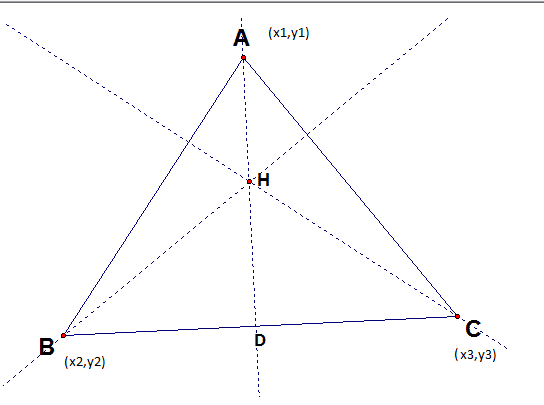# Triangle altitude's concurrency by symmetry

## Homework Statement

in the proof of triangle altitudes concurrency , i have found the equation of the Altitude AD,
x(x2-x3)+y(y2-y3)-x1(x2-x3)-y1(y2-y3)## The Attempt at a Solution

In the book other altitude equations are written by symmetry,

how is the idea of symmetry is used here ? , .

#### Attachments

Last edited by a moderator:

FactChecker
Gold Member
The first thing you must learn is to be more careful. The problem statement looks incomplete. What is D, x, y, x1, x2, x3, y1, y2, y3?

Did the book say "symmetry"? I don't see any symmetry, but you may have left out some important parts of the problem statement. Otherwise, "symmetry" is probably the wrong word to use. A better word might be "similarly".

The first thing you must learn is to be more careful. The problem statement looks incomplete. What is D, x, y, x1, x2, x3, y1, y2, y3?

Did the book say "symmetry"? I don't see any symmetry, but you may have left out some important parts of the problem statement. Otherwise, "symmetry" is probably the wrong word to use. A better word might be "similarly".

i have now uploaded the image of triangle ,

SammyS
Staff Emeritus
Homework Helper
Gold Member

## Homework Statement

in the proof of triangle altitudes concurrency , i have found the equation of the Altitude AD,
x(x2-x3)+y(y2-y3)-x1(x2-x3)-y1(y2-y3)
View attachment 219067

## The Attempt at a Solution

In the book other altitude equations are written by symmetry,

how is the idea of symmetry is used here ? , .

x(x2-x3)+y(y2-y3)-x1(x2-x3)-y1(y2-y3)

is a mathematical expression, not an equation. It has no equal sign.

Did you mean to set this expression equal to zero ?

FactChecker
Gold Member
The diagram I see now is better. Did the book say anything else about the triangle? Is it equilateral or isosceles?

•alijan kk
LCKurtz
Homework Helper
Gold Member
@alijan kk: As others have pointed out, it isn't "symmetry", but here is what your author is using. In your figure, if you rotate the labels counterclockwise you will have replaced the subscripts 1 by 2, 2 by 3, and 3 by 1.Then the same steps on the points A, B, and C that were used to get your original expression will give an answer you can get by replacing 1 by 2, 2 by 3, and 3 by 1 in your original expression. Then rotate the subscripts one more time for the third equation. (It has already been pointed out to you that you haven't written equations).

•alijan kk
SammyS
Staff Emeritus